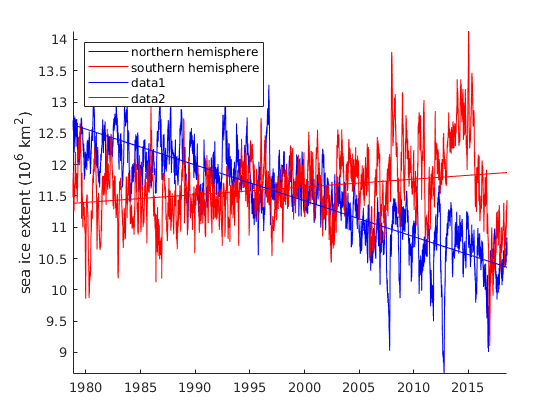# deseason documentation

deseason removes the seasonal (aka annual) cycle of variability from a time series.

See also: season, climatology, sinefit, sineval, and sinefit_bootstrap.

Back to Climate Data Tools Contents

## Syntax

```Ads = deseason(A,t)

## Description

Ads = deseason(A,t) removes the typical seasonal (aka annual) cycle from the time series A corresponding to times t in datenum format. If t is daily, outputs ts is 1 to 366 and As will contain average values for each of the 366 days of the year. If inputs are monthly, ts is 1:12 and As will contain average values for each of the 12 months of the year.

Ads = deseason(...,'daily') specifies directly that inputs are daily resolution. The deseason function will typically figure this out automatically, but if you have large missing gaps in your data you may wish to ensure correct results by specifying daily.

Ads = deseason(...,'monthly') as above, but forces monthly solution.

Ads = deseason(...,'detrend',DetrendOption) specifies a baseline relative to which seasonal anomalies are determined. Options are 'linear', 'quadratic', or 'none'. By default, anomalies are calculated after removing the linear least squares trend, but if, for example, warming is strongly nonlinear, you may prefer the 'quadratic' option. NOTE: The deseason function does NOT return detrended data. Rather, detrending is only performed to determine the seasonal cycle. Default is 'linear'.

Ads = deseason(...,'dim',dimension) specifies a dimension along which to assess seasons. By default, if A is 1D, seasonal cycle is returned along the nonsingleton dimension; if A is 2D, deseason is performed along dimension 1 (time marches down the rows); if A is 3D, deseason is performed along dimension 3.

## Example: Sea ice extent

Consider this sample sea ice extent data:

```load seaice_extent

figure
plot(t,extent_N,'b')
hold on
plot(t,extent_S,'r')

axis tight
box off
legend('northern hemisphere','southern hemisphere','location','northwest')
ylabel ' sea ice extent (10^6 km^2) '
```As you can see, sea ice extent variability is overwhelmingly dominated by seasonal cycles. Let's deseason each time series to get a better idea of what's happening interannually:

```north_ds = deseason(extent_N,t);
south_ds = deseason(extent_S,t);

plot(t,north_ds,'b')
plot(t,south_ds,'r')
```Let's make a new figure with just the detrended data, and use polyplot to add trendlines:

```figure
plot(t,north_ds,'b')
hold on
plot(t,south_ds,'r')
axis tight
box off
legend('northern hemisphere','southern hemisphere','location','northwest')
ylabel ' sea ice extent (10^6 km^2) '

% Overlay a trend line:
polyplot(t,north_ds,1,'b')
polyplot(t,south_ds,1,'r')
```The plot above shows that throughout the satellite epoch, northern hemisphere sea ice extent has declined while southern hemisphere sea ice extent has increased. But it might be tough to see which hemisphere is winning. An easy way to see what's happening globally is to simply add northern and southern hemisphere sea ice extents:

```figure
plot(t,north_ds+south_ds,'k')
hold on
polyplot(t,north_ds+south_ds,1,'k','linewidth',2)
axis tight
box off
ylabel ' global sea ice extent (10^6 km^2) '
```The plot above makes it clear that Antarctic sea ice gains are not keeping up with Arctic sea ice losses.

We can quantify the global sea ice trend with the trend function. The trend function calculates the linear trend relative to the units you give it, and we're working with datenum units which are days, so we'll multiply by 365.25*10 to get the sea ice trend in "per decade" units, and multiply by 1e6 to get units in km^2:

```trend(extent_S+extent_N,datenum(t))*365.25*10*1e6
```
```ans =
-4.5833e+05
```

That tells us over the satellite record, we've been losing almost half a million square kilometers of sea ice per decade, globally.

## How this function works

The deseason function simply works like this:

`Ads = A - season(A,t);`

You will find a description of how the season function calculates the seasonal signal here.

## Seasons vs Climatology

CDT has a function called season and another function called climatology. The only difference is that the output of climatology includes the mean of the variable, whereas the output of season will always have a 0 mean value. Accordingly, the deseason function removes the seasonal component of variability while preserving the overall mean and trends.

In general, CDT assumes that a multi-year record of a variable sampled at subannual resolution can described by

`y = y_0 + y_tr + y_season + y_var + y_noise`

where

• y_0 is the long-term mean,
• y_trend is the long-term trend,
• y_season is the typical seasonal anomaly, which the season function obtains after detrending and removing the mean,
• y_var represents interannual variability, and
• y_noise is everything else

In this model,

`y_climatology = y_0 + y_season`

## Other ways to define seasonality

For yet another way to define seasonality, see sinefit, sineval, and sinefit_bootstrap.

## Author Info

This function was written by Chad A. Greene of the University of Texas Institute for Geophysics (UTIG), July 2017.